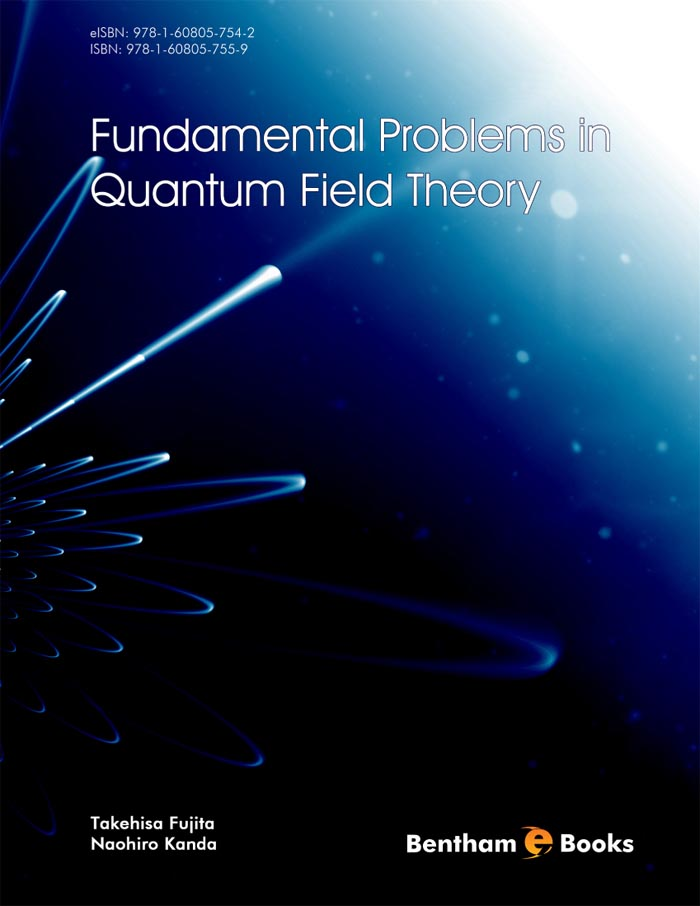### Quantum Electrodynamics

#### Abstract

Here, we present the calculations of the self-energy of photon and electron. First the renormalization scheme of photon is discussed in connection with the triangle diagrams which have no divergence at all, and therefore the self-energy of photon is not related to any physical observables. Then, we discuss the renormalization scheme of fermion self-energy term which is still connected with the vertex correction in QED. Also, we discuss briefly the physics of Lamb shifts which should be treated in detail in chapter 7. Then, we present the calculation of the photonphoton scattering cross section and some possible experiments on the photon-photon cross section. Finally, the problem of the chiral anomaly equation is discussed, and we see that there are neither anomaly equation nor the violation of the axial current conservation in physical world.

Total Pages: 35-71 (37)